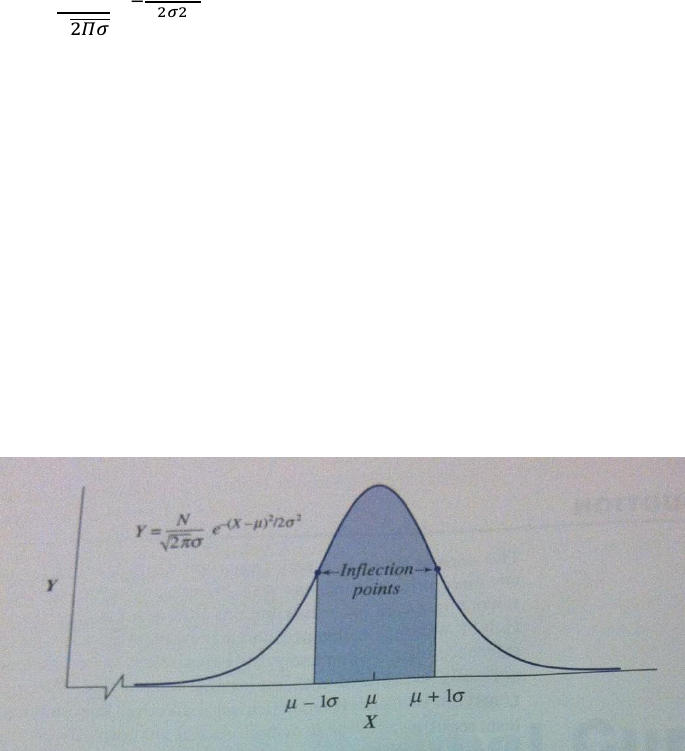# PSY201H1 Chapter Notes - Chapter 5: Percentile Rank, Standard Deviation, Standard Score

16 views3 pages
Page:
of 3Understanding Statistics Chapter 5
Normal Curve
A theoretical distribution of population scores
A bell-shaped curve that is described by this equation:
Equation of a normal curve:
Y=

Y= frequency of the given value of X*
X= any score in the distribution
μ= mean of the distribution
σ= standard deviation of the distribution
N= total frequency of the distribution
∏= a constant of 3.1416
e= a constant of 2.7183
This makes a bell-shaped curve
At the bell curve, there are 2 inflection points where the curves go from being
convex downward to convex upward, and are at 1 standard deviation from the mean
(μ+1σ and μ-1σ)
Theoretically, it never reaches the horizontal axis, and therefore is asymptotic to it
Area under the Normal Curve
The area between the inflection points accounts for 50% of the area
Between the mean and 1 standard deviation point = 34.13%
Between the 1st and 2nd standard deviation points = 13.59
Standard Scores, or “Z-Scores”
To make a raw score meaningful, you need to find the percentile rank
Remember, the percentile rank is the percentage of scores that are below the given
score)
If the score is above the μ, then add 50% to account for the half of the scores below the
mean, and find the percentage between the mean and the standard deviation
In the example in the book, the mean is 100 for an IQ test and you score 132, which
is exactly 2 standard deviations from the mean. So, remember the percentages
named above:
Mean 1σ = 34.13
1σ2σ = 13.59
Add these = 47.72%
Now remember to add the 50% from the other side of the bell curve, and you
end up with 97.72%
This means that you are smarter than 97.72% of the population set!!
We had to find out how many standard deviations the raw score was from the mean,
and in turn we found out the standard score, or ‘z-score’
Equation:
This is the z-score for the population data: z= 
This is the z-score for sample data: z= 
For the previous example: z=
 = 2.00
Z-scores are called standard scores because they are expressed relative to a
distribution mean of 0 and a standard deviation of 1
Z-scores also allow you to compare scores in different distributions with different
units of distribution. For example, saying that an IQ of 132 is 97.72% higher than the
rest of the population and comparing it to a rat’s weight of 340 grams being 97.72%
higher than the rest of the rats allows you to correlate things from completely
different contexts
Recap!
First, find the SS
SS= ΣX2-
So, take the sum of each of the raw scores squared, minus (the squared
sum of all of the scores/the amount of scores)
Once you have the SS, plug it into this equation:
s=

Basically, the answer you got/the amount of scores minus one
Then, you take the square root of that and you have your z-score!!
Characteristics of z-scores
They have the same shape as the set of the raw scores
The mean of the z-scores will always equal zero (μz=0)
The standard deviation of z-scores always equals one (σz=1)
Finding the Area, Given the Raw Score
Find z
z = 
Once you find z, refer to the table that contains the z score
Column B = proportion of the area between the z you found and the mean
Column C = the proportion of the area that exists beyond the z-score
Be sure to draw out a bell curve and place the z-score and mean on it. This will help you
to visualise where B and C go
Use B and C to calculate the percentage of scores above or below the z-score while
using the data from the table
Finding the Raw Score, Given the Area
Just work backwards!!
Use the given percentage to determine the C score (100-given percent) and look at teh
corresponding value in the C Column on the table
Then, plug in the values you have and work backwards